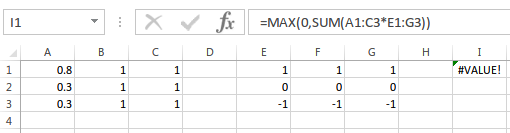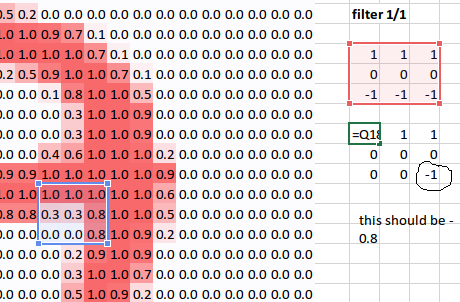# Lesson 3: Excel Convolution Kernel Layer 1 formula

I was playing around with excel and tried to replicate the first layer formula, but instead of getting a value like @jeremy did, I get a `#VALUE` error, like so:Can anyone please tell me what I am doing wrong? I am a noob at excel so forgive me if this is something really dumb. Thanks

It’s an array formula, which means you have to hit ctrl+shift+enter rather than just enter.

1 Like

Ah, I see. Thank you.

@jeremy Ok, One final question. Does excel automatically rounds off decimal values because I noticed that whn I did the calculation(matrix element wise multiplication) by hand, they match with excel’s only if I round them off. Am I correct or is there something else to the calculation. For eg in the picture the value is -1 instead of being -0.8: